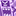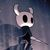# New to Qlik Sense

If you’re new to Qlik Sense, start with this Discussion Board and get up-to-speed quickly.

Announcements
Our May 2021 end-to-end product release from Data Integration to Data Analytics is out! READ DETAILS
cancel
Showing results for
Search instead for
Did you mean:Contributor II

## Count(If vs Count({<>}

Hello community,

I have a big question since it is drawling some big discrepancies in my thought process.

I am trying to count with a condition...

I am under the impression I should be able to do this with either of the two lines of code listed below; however, I get two different results. So my question is how do the two lines of code act differently? Or is there something being done wrong on the back end that would have caused this.

Count({<event_type={33,34}>}od_id)

vs

Count(if(event_type=33, od_id) or if(event_type=34, od_id) )

Note I have tried different variations of these.

I have also tried to diagnose this with only one event_type and still get two different results.

Count({<event_type={33}>}od_id)      //will produce 102.1K

vs

Count(if(event_type=33, od_id) )        // will produce 173.6K

Clarification on how these two formulas are working differently would be greatly appreciated.

Thanks!

Labels (1)
• ### Count clarification

6 RepliesMVP

Are the two fields coming from different tables in the data model of the app?Contributor II
Author

The fields are from two separate tables.Partner

Not sure it helps (and you said you tried variations on the formulae), but I do the if counts the other way around :

``if(event_type=33, count(od_id))``

or

``if(event_type=33, count(od_id), if(event_type=34, count(od_id)))``Partner
``Count({<>}``

aggregates over the set that you define there, disregarding any selection or filters you may have currently applied.

If you're showing data that is not the entire set you're loading in your table, the two functions will show different results - and that is normal behaviour because they aren't the same.

For instance, I have a graph that shows the average value for the 3 months prior to every month ; so for month X it averages over the months X-2, X-1 and X. When I select a month though, the X-2 and X-1 values are filtered out and the average is then only over month X ; if I use

``RangeAvg(Above(Sum({<Month=>}[Data]), 0, 3))``

instead of

``RangeAvg(Above(Sum([Data]), 0, 3))``

the average is still calculated even when only one month is selected.Contributor II
Author

Do you use this method in the front end? or the back end? I tried your code in the front end and just get a "-". Note that the fields are from two tables linked by a different IDPartner

I use this front-end. Perhaps try this :

``if(event_type=33, count(od_id), if(event_type=34, count(od_id)), 0)``

It is possible that there is a mix of types that results in undefined data, hence the ' - '